### Astrophysics

Everything we know about the universe, we learned by sifting through bits and pieces of energy sent in our direction from far-away sources. Detecting this energy is what allows humans to unravel the mysteries of the universe while our feet (mostly) remain on the ground.Radiation—traveling particles of matter and light—brings information about the universe to us. Radiation is a by-product of atomic and nuclear processes that carries away excess energy. In this quiz we will explore how radiation is produced, characterized and detected.

Which do you think would not be considered radiation?

The typically high speeds of radiation make it particularly useful for studying astronomical objects separated by astronomical distances. Visible light (and all types of electromagnetic radiation) travels through empty space at a rate of $c=\SI[per-mode=symbol]{2.998e8}{\meter\per\second}.$

Large-scale manufacturing of incandescent light bulbs began about 100 years ago, heralding the age of cheap, safe artificial lighting on Earth.Some of the light escaped through Earth's atmosphere and is today traveling through space. How far from our solar system is the light emitted $100$ years ago today?

Using a telescope, you observe light reflected from Eris, a dwarf planet in orbit beyond Pluto.

By the time the light from Eris enters your telescope on Earth, Eris has moved away from the position where you see it. How long ago $($in $\si{min})$ was Eris in the position where you observe it?


Details and Assumptions:

• Assume Eris is $\SI{50}{au}$ from Earth when you observe it. $\SI{1}{au}=\SI{1.5e11}{\meter}.$
• In practice, most people round the speed of light to $\SI[per-mode=symbol]{3.0e8}{\meter\per\second}.$
• In the next chapter, we will take a detailed look at observations astronomers make in order to work out the distances to objects within and outside our solar system.

All electromagnetic radiation moves away from a radiating source at the same speed, but that does not mean all light is identical.

Light can have a range of wavelengths; different wavelengths of light have different effects on matter. Wavelength is represented by the symbol $\lambda.$ Altogether, the continuum of wavelengths is called the electromagnetic (EM) spectrum. Light can have wavelengths outside of the range we can see; in fact, visible light is just a sliver of the entire EM spectrum.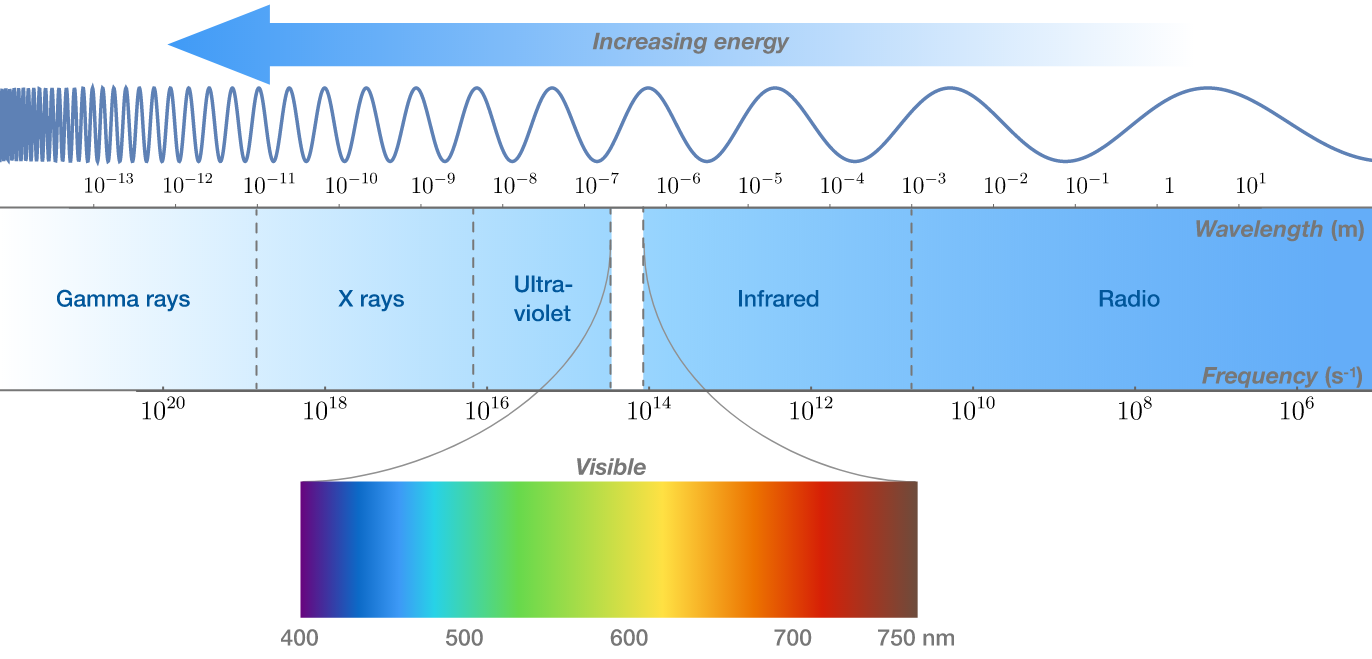All radiation is characterized by wavelength, with shorter wavelength indicating higher energy. In the $20^\text{th}$ century, physicists measured wavelengths of matter particle radiation, like electrons. This is the starting point of quantum mechanics. We will come back to wave properties of matter in a later quiz.

Types of EM radiation (x-rays, ultraviolet, infrared, visible, and radio waves) are distinguished by their wavelengths, but they are just as often classified by frequency $f,$ the number of waves passing a point per second. Because wavelength and frequency are used interchangeably, we should learn how to "convert" between them.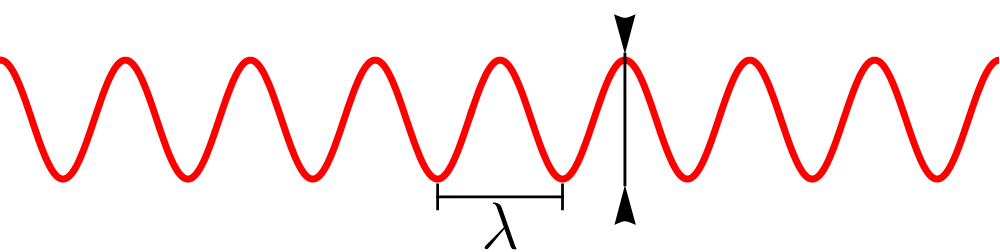What relationship tells us the frequency of a wave that has wavelength $\lambda$ which is traveling with speed $c?$


Details and Hint:

• $c$ is the speed of light.
• It may help to know that the standard unit of $\lambda$ is $\si{\meter}$ and the standard unit of $f$ is $\SI[per-mode=symbol]{1}{\per\second}.$

Although we have characterized EM radiation on a spectrum of wavelengths, the energy carried by EM radiation is delivered by massless particles called photons. Experiments show that the amount of energy carried by each photon is proportional to the radiation frequency: $E_\text{photon}=hf,$ where $h$ is called Planck's constant and is equal to $h=\SI{6.63e-34}{\joule\second}.$

The tungsten filament in an incandescent light bulb emits visible light with wavelength $\SI{500}{\nano\meter}=\SI{5e-7}{\meter}$ $($since one nanometer is $\SI{10^{-9}}{\meter}).$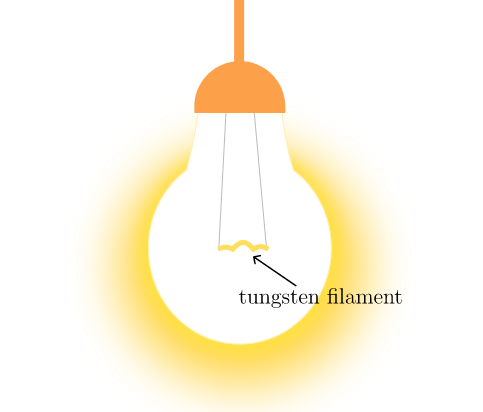About how much energy does a typical visible photon carry?

A light bulb filament does not emit EM radiation at a single wavelength and frequency. Rather, it emits according to a distribution of wavelengths known as the tungsten filament's thermal emission spectrum.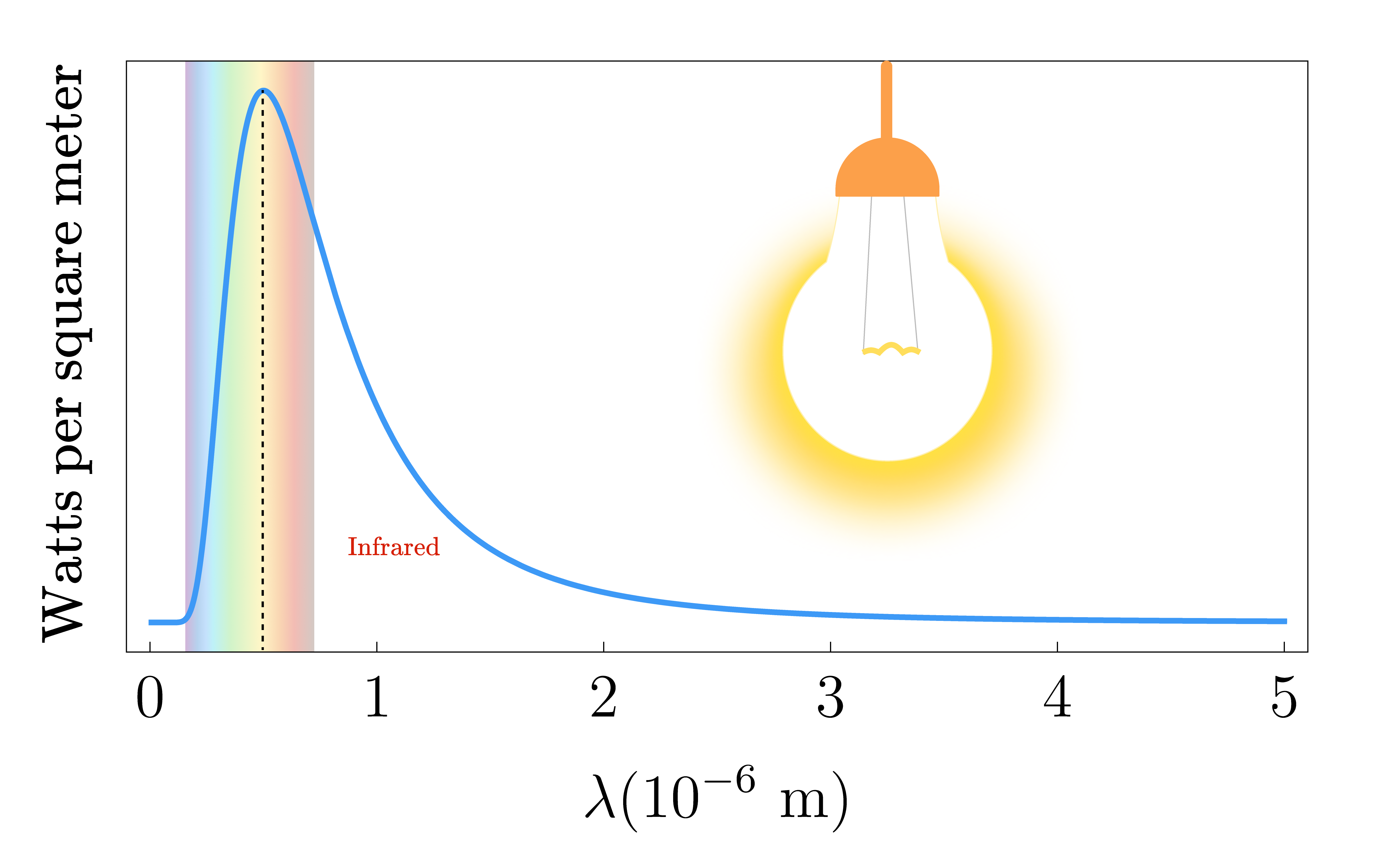Every object has a unique emission spectrum that depends on its atomic structure and temperature, but all known emission spectra have a peak wavelength that depends in a predictable way on temperature.

You can see that the peak of tungsten's spectrum is near yellow in the visible band. Suppose we supplied more voltage to the light bulb so that the filament's temperature increases. How would you expect the peak of the emission spectrum to change?

All matter, hot or cold, emits EM radiation—sometimes called thermal or blackbody radiation. In response to changes in temperature, an emission spectrum's maximum changes in a predictable way.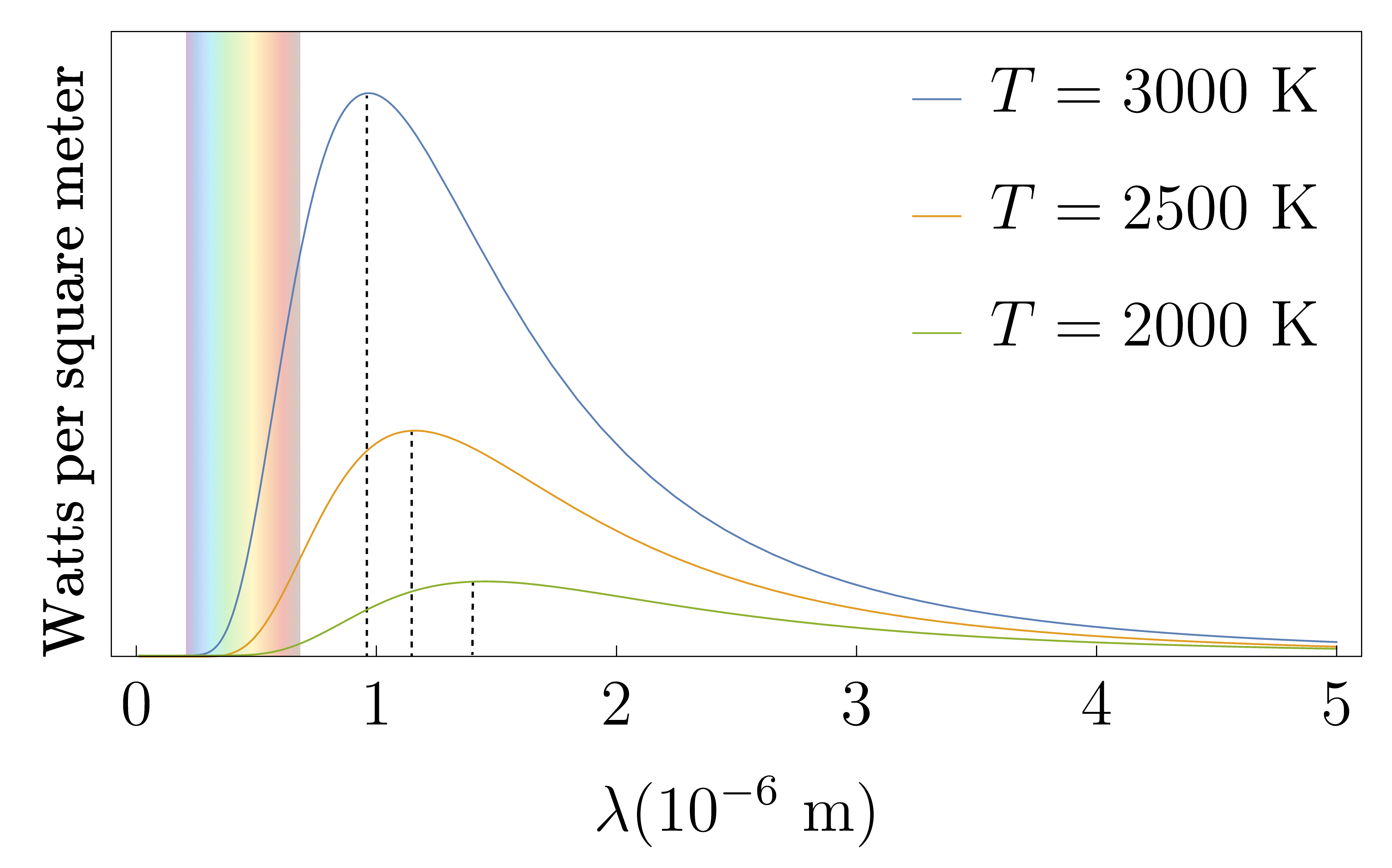The colors of the visible spectrum are shown in a band around $\SI{0.5e-6}{\meter}.$ Objects below temperatures of several thousand Kelvin emit mostly at longer wavelengths, which is why they don't glow like hotter objects.

The peak wavelength of the thermal emission spectrum $\lambda_\textrm{max}$ (indicated by dotted lines for the three emission spectra above) is inversely proportional to the temperature $T$: $\lambda_\textrm{max} = \frac{P_0}{T}$

where the constant $P_0=\SI{0.0029}{\meter\cdot\kelvin}.$ This is called Wien's displacement law.

How hot is a tungsten filament when its peak emitted wavelength is in the yellow band of the visible spectrum?


Details and Assumptions:

• Use Wien's displacement law and estimate the wavelength of the yellow band from the diagram.

Peak wavelength of a radiating body is an easy quantity to measure, no matter how far the collected light has traveled to us. For example, the emission spectrum of the Sun is plotted below.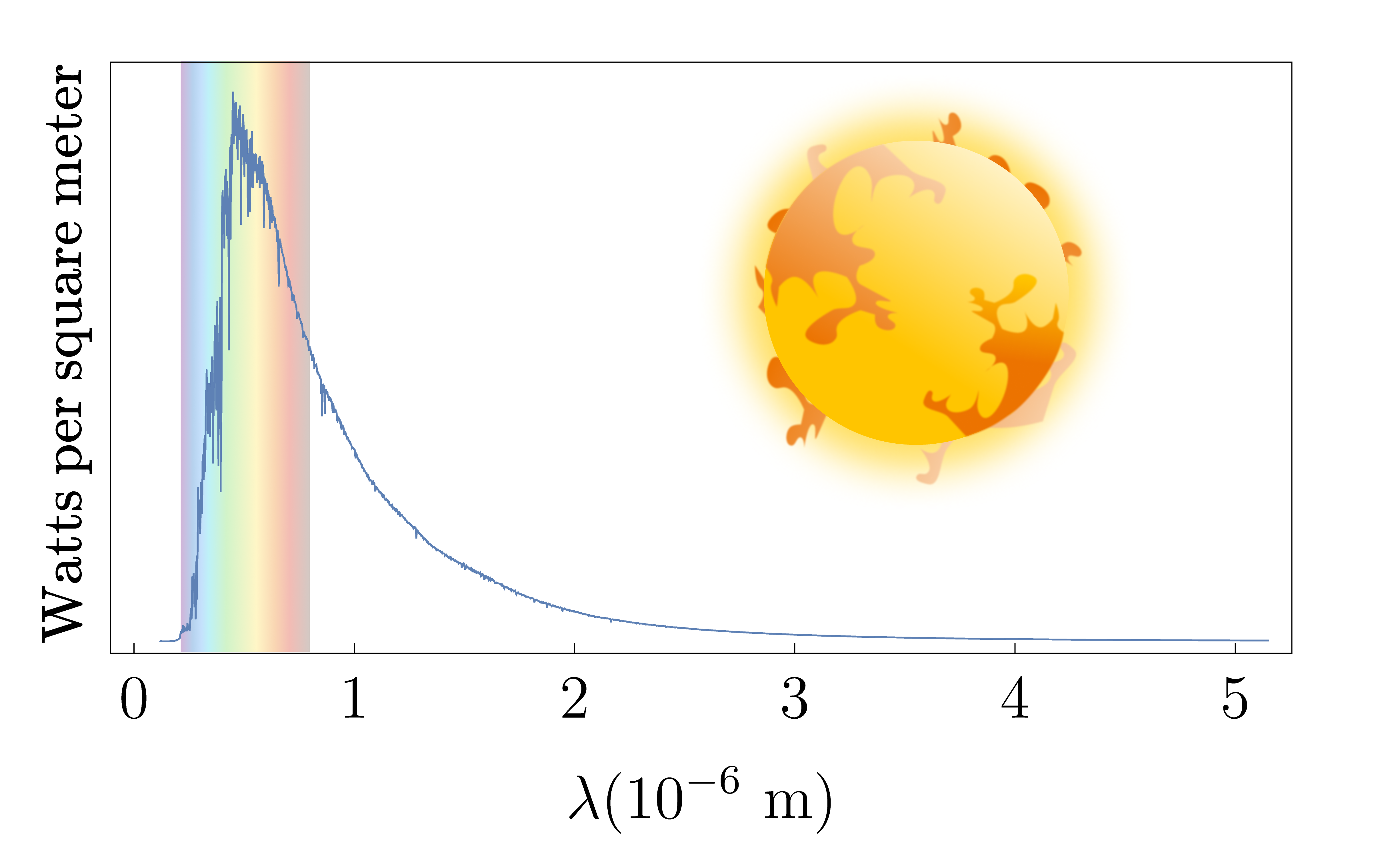The Sun has a peak wavelength around $\SI{500}{\nano\meter}$—same as the filament in the previous question. Thus, we can estimate that the Sun's surface temperature is about the same as a tungsten filament, $\SI{5800}{\kelvin}.$ In fact, Wien's displacement law can be used to estimate the surface temperature of any nearby star.

All matter emits EM radiation in different regions of the electromagnetic spectrum, depending on whether it is hot or cold. Astronomers can collect and analyze not only the visible light from stars we can see in the night sky but also light across the EM spectrum: infrared, radio, UV, x-rays, etc. In the next quiz, we will see how peak wavelengths are distributed for clusters of stars in our galaxy when we introduce the famed Hertzsprung-Russell diagram.